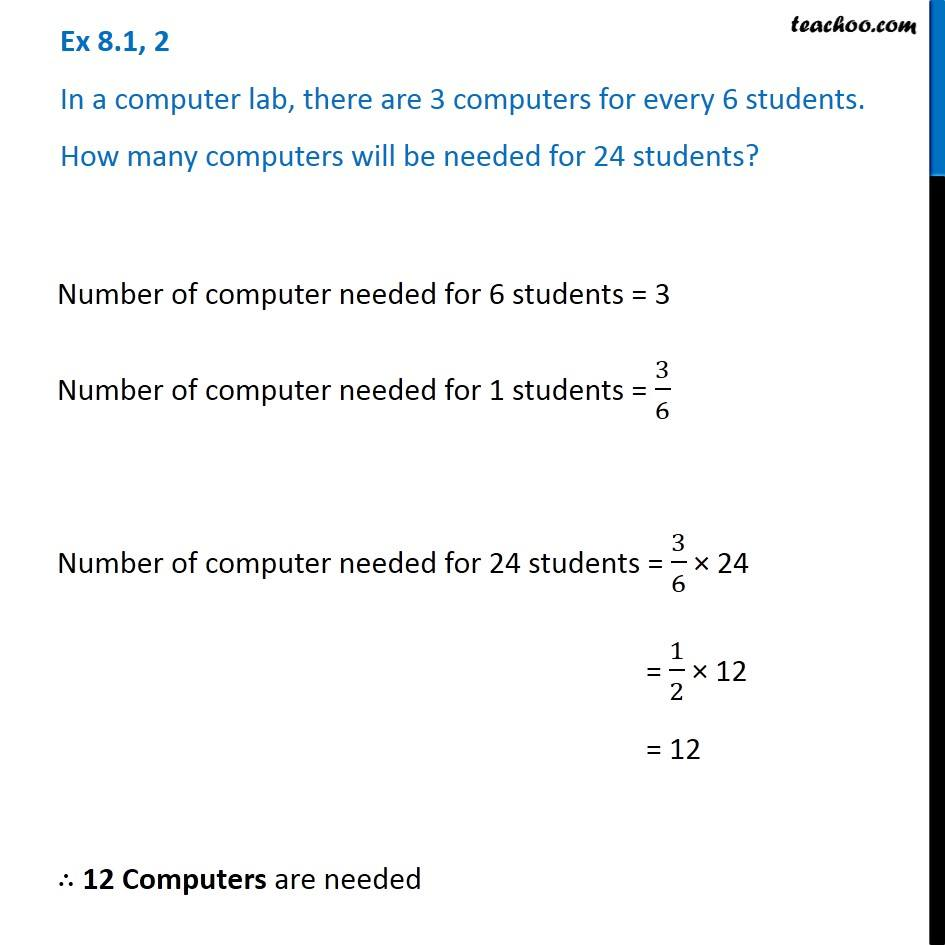Unitary Method

Chapter 8 Class 7 Comparing Quantities
Concept wiseIntroducing your new favourite teacher - Teachoo Black, at only ₹83 per month

### Transcript

Ex 8.1, 2 In a computer lab, there are 3 computers for every 6 students. How many computers will be needed for 24 students? Number of computer needed for 6 students = 3 Number of computer needed for 1 students = 3/6 Number of computer needed for 24 students = 3/6 × 24 = 1/2 × 12 = 12 ∴ 12 Computers are needed Ex 8.1, 2 In a computer lab, there are 3 computers for every 6 students. How many computers will be needed for 24 students? Number of computer needed for 6 students = 3 Number of computer needed for 1 students = 3/6 Number of computer needed for 24 students = 3/6 × 24 = 1/2 × 12 = 12 ∴ 12 Computers are needed